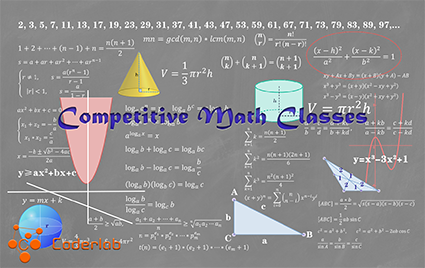## Competitive Math Classes

Director

Instructor

Instructor

Instructor

##### Anujun kandasamy#### Competitive Math Classes

Timing: Wednesday night 6:30 - 8:00 PM, Weekly for 10 weeks

Date: Starting from July 6th 2022

Workshop Location: Zoom online class

This course provides students with an organized preparation for the upcoming Grade 9 Contest. We will review past Grade 9 math contests by providing in-depth explanations, formulas, topics and solutions to a selection of problems, and answering any questions about Math contests. After the first day of class, we will expect you to finish your assigned homework and engage in the classroom discussion during the next class.

These Math classes will help students to develop a deeper rooted understanding of methodologies in the high school Math contest.

Homework ( Resources originated from University of Waterloo CEMC).

We will try to cover the following topics as much as we can in the classes.

Number Theory

• Calculation and properties of congruence
• Euler's function
• Fermat's little theorem
• Floor function
• Odd and even numbers
• Number of factors
• Conversion between different numerical systems

Functions and Polynomials

• Abstract functions
• Symmetry
• Vieta 's theorem of high-degree polynomials
• Remainder theorem
• Factor theorem

Sequences and Series

• Recursive expressions
• Fibonacci sequence
• Sum of sequences
• Geometrical series

Algebra

• Binomial theorem
• Factorization and equation
• Basic inequalities

Geometry

• Sine rule and cosine rule
• Similar triangles
• Heron's formula
• Properties of circle
• 3-d geometry

Analytic Geometry

• Equation of straight lines
• Distance between a point and a line
• Equation of circle

Permutation and Combination

• Principle of inclusion and exclusion
• Principle of multiplication
• Models of permutation and combination

Probability and Statistics

• Conditional probability
• Complex probability problems
• Basic statistical concepts

Logic and Reasoning

• Word problems
• Comprehension of complex problems
• Conversion of problems

Logarithm

• Rules of logarithm
• Logarithm and exponent
• Equation of logarithm

Complex Numbers and Trigonometry

• Trigonometrical identities
• Maximum and minimum of trig functions
• Polar form and exponential form of complex numbers
• De Moivre's theorem

r